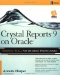# Appendix B Functions

This appendix contains the code listings for functions used in the book. A script containing the PL/SQL functions is available at Appendix BFunctions.sql.

### Reproducing Crystal Functions

Some Crystal Reports functions have no equivalent in Oracle. This section contains the code necessary to create those Oracle functions. Note that no exception handling is performed in these functions.

### CRMOD

CRMOD is an Oracle equivalent for the Crystal MOD function. The existing Oracle MOD function does not behave identically to Crystal’s version, but this version will:

```CREATE OR REPLACE FUNCTION CRMOD
(XVal IN NUMBER,
YVal IN NUMBER)
RETURN NUMBER
AS
BEGIN
RETURN MOD(ROUND(XVal),ROUND(YVal));
END;```

### INT_DIV

INT_DIV can be used instead of the Crystal integer division operator ():

```CREATE OR REPLACE FUNCTION INT_DIV
(Numerator IN NUMBER,
Denominator IN NUMBER)
RETURN NUMBER
AS
BEGIN
RETURN FLOOR(ROUND(Numerator)/ROUND(Denominator));
END;```

### PERCENT

The PL/SQL function PERCENT is a replacement for the Crystal (%) operator:

```CREATE OR REPLACE FUNCTION PERCENT
(Numerator IN NUMBER,
Denominator IN NUMBER)
RETURN NUMBER
AS
BEGIN
RETURN 100*(Numerator/Denominator);
END;
```

### Other Functions

Several conversion functions are beneficial when using Crystal Reports with Oracle. In the future, they may become obsolete as Crystal incorporates native support for Oracle 9i’s new datatypes.

### TIMESTAMP_TO_DATE

TIMESTAMP_TO_DATE converts a timestamp to a date:

```CREATE OR REPLACE FUNCTION TIMESTAMP_TO_DATE
(TimestampVar IN TIMESTAMP)
RETURN DATE
AS
BEGIN
'DD-MON-YYYY HH24:MI:SS');
END;```

### INTERVAL_TO_YEARS

INTERVAL_TO_YEARS converts a YEAR TO MONTH interval variable to a number of years, including the number of months as a fractional portion of a year:

```CREATE OR REPLACE FUNCTION INTERVAL_TO_YEARS
(IntervalVar IN INTERVAL YEAR TO MONTH)
RETURN NUMBER
AS
BEGIN
RETURN EXTRACT(YEAR FROM IntervalVar)
+EXTRACT(MONTH FROM IntervalVar)/12;
END;
```

### INTERVAL_TO_MONTHS

INTERVAL_TO_MONTHS converts a YEAR TO MONTH interval variable to a number of months:

```CREATE OR REPLACE FUNCTION INTERVAL_TO_MONTHS
(IntervalVar IN INTERVAL YEAR TO MONTH)
RETURN NUMBER
AS
BEGIN
RETURN EXTRACT(YEAR FROM IntervalVar)*12
+EXTRACT(MONTH FROM IntervalVar);
END;```

### INTERVAL_TO_DAYS

INTERVAL_TO_DAYS converts a DAY TO SECOND interval to a number of days including hours, minutes, and seconds as a fractional portion of a day:

```CREATE OR REPLACE FUNCTION INTERVAL_TO_DAYS
(IntervalVar IN INTERVAL DAY TO SECOND)
RETURN NUMBER
AS
BEGIN
RETURN EXTRACT(DAY FROM IntervalVar)
+EXTRACT(HOUR FROM IntervalVar)/24
+EXTRACT(MINUTE FROM IntervalVar)/(24*60)
+EXTRACT(SECOND FROM IntervalVar)/(24*60*60);
END;```

### INTERVAL_TO_HOURS

INTERVAL_TO_HOURS converts a DAY TO SECOND interval to a number of hours, which may be more than 24 and includes minutes and seconds as a fractional portion of an hour:

```CREATE OR REPLACE FUNCTION INTERVAL_TO_HOURS
(IntervalVar IN INTERVAL DAY TO SECOND)
RETURN NUMBER
AS
BEGIN
RETURN EXTRACT(DAY FROM IntervalVar)*24
+EXTRACT(HOUR FROM IntervalVar)
+EXTRACT(MINUTE FROM IntervalVar)/60
+EXTRACT(SECOND FROM IntervalVar)/(60*60);
END;
```

### INTERVAL_TO_MINUTES

INTERVAL_TO_MINUTES converts a DAY TO SECOND interval to a number of minutes, which may be more than 60 and includes seconds as a fractional portion of a minute:

```CREATE OR REPLACE FUNCTION INTERVAL_TO_MINUTES
(IntervalVar IN INTERVAL DAY TO SECOND)
RETURN NUMBER
AS
BEGIN
RETURN EXTRACT(DAY FROM IntervalVar)*24*60
+EXTRACT(HOUR FROM IntervalVar)*60
+EXTRACT(MINUTE FROM IntervalVar)
+EXTRACT(SECOND FROM IntervalVar)/60;
END;```

### INTERVAL_TO_SECONDS

INTERVAL_TO_SECONDS converts a DAY TO SECOND interval to a number of seconds:

```CREATE OR REPLACE FUNCTION INTERVAL_TO_SECONDS
(IntervalVar IN INTERVAL DAY TO SECOND)
RETURN NUMBER
AS
BEGIN
RETURN EXTRACT(DAY FROM IntervalVar)*60*60*24
+EXTRACT(HOUR FROM IntervalVar)*60*60
+EXTRACT(MINUTE FROM IntervalVar)*60
+EXTRACT(SECOND FROM IntervalVar);
END;```Crystal Reports 9 on Oracle (Database Professionals)
ISBN: B003D7JY9U
EAN: N/A
Year: 2005
Pages: 101